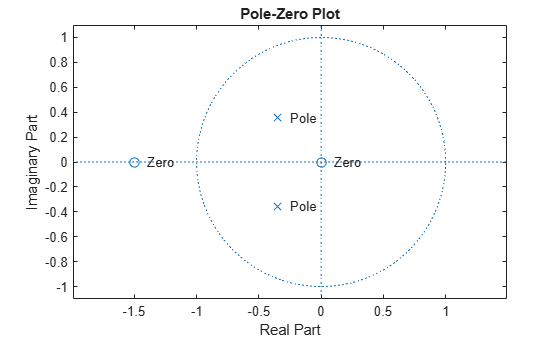# tf2zp

Convert transfer function filter parameters to zero-pole-gain form

## Syntax

``[z,p,k] = tf2zp(b,a)``

## Description

example

````[z,p,k] = tf2zp(b,a)` finds the matrix of zeros `z`, the vector of poles `p`, and the associated vector of gains `k` from the transfer function parameters `b` and `a`. The function converts a polynomial transfer-function representation $H\left(s\right)=\frac{B\left(s\right)}{A\left(s\right)}=\frac{{b}_{1}{s}^{n-1}+\cdots +{b}_{n-1}s+{b}_{n}}{{a}_{1}{s}^{m-1}+\cdots +{a}_{m-1}s+{a}_{m}}$of a single-input/multi-output (SIMO) continuous-time system to a factored transfer function form $H\left(s\right)=\frac{Z\left(s\right)}{P\left(s\right)}=k\frac{\left(s-{z}_{1}\right)\left(s-{z}_{2}\right)\cdots \left(s-{z}_{m}\right)}{\left(s-{p}_{1}\right)\left(s-{p}_{2}\right)\cdots \left(s-{p}_{n}\right)}.$ NoteUse `tf2zp` when working with positive powers (s2 + s + 1), such as in continuous-time transfer functions. A similar function, `tf2zpk`, is more useful when working with transfer functions expressed in inverse powers (1 + z–1 + z–2). ```

## Examples

collapse all

Generate a system with the following transfer function.

`$H\left(s\right)=\frac{2{s}^{2}+3s}{{s}^{2}+\frac{1}{\sqrt{2}}s+\frac{1}{4}}=\frac{2\phantom{\rule{0.16666666666666666em}{0ex}}\left(s-0\right)\phantom{\rule{0.16666666666666666em}{0ex}}\left(s-\left(-\frac{3}{2}\right)\right)}{\left(s-\frac{-1}{2\sqrt{2}}\left(1-j\right)\right)\phantom{\rule{0.16666666666666666em}{0ex}}\left(s-\frac{-1}{2\sqrt{2}}\left(1+j\right)\right)}$`

Find the zeros, poles, and gain of the system. Use `eqtflength` to ensure the numerator and denominator have the same length.

```b = [2 3]; a = [1 1/sqrt(2) 1/4]; [b,a] = eqtflength(b,a); [z,p,k] = tf2zp(b,a)```
```z = 2×1 0 -1.5000 ```
```p = 2×1 complex -0.3536 + 0.3536i -0.3536 - 0.3536i ```
```k = 2 ```

Plot the poles and zeros to verify that they are in the expected locations.

```zplane(b,a) text(real(z)+0.1,imag(z),"Zero") text(real(p)+0.1,imag(p),"Pole")```## Input Arguments

collapse all

Transfer function numerator coefficients, specified as a vector or matrix. If `b` is a matrix, then each row of `b` corresponds to an output of the system. `b` contains the coefficients in descending powers of s. The number of columns of `b` must be less than or equal to the length of `a`.

Data Types: `single` | `double`

Transfer function denominator coefficients, specified as a vector. `a` contains the coefficients in descending powers of s.

Data Types: `single` | `double`

## Output Arguments

collapse all

Zeros of the system, returned as a matrix. `z` contains the numerator zeros in its columns. `z` has as many columns as there are outputs.

Poles of the system, returned as a column vector. `p` contains the pole locations of the denominator coefficients of the transfer function.

Gains of the system, returned as a column vector. `k` contains the gains for each numerator transfer function.

## Version History

Introduced before R2006a